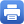Columbia Home
March 4: Horng-Tzer Yau (Harvard)

Title: A dynamical approach to universality in probability theory with applications in random matrices

Abstract:

The eigenvalue and eigenvector behaviors are fundamental questions in many areas of sciences.
In this lecture, we will review recent results concerning eigenvalue and eigenvector statistics for random matrix and random graph models, including random $d$-regular graphs and random matrices with fat tails.
A separate question concerns the rate of relaxation to equilibrium of the matrix Brownian motions defined by the flow $t \to B(t)$ where $B(t)$ is a symmetric matrix with Brownian motions as matrix elements.
We will explain that a resolution of this dynamical question has a profound implication for the analysis of eigenvalue and eigenvector statistics of random matrices.

Wednesday, March 4, 4:30 – 5:30 p.m.

Mathematics 520
Tea will be served at 4:00 p.m.Print this page